• 对结构体数组学生信息进行输入输出
千次阅读
2021-10-17 15:08:26

#include<stdio.h>
struct student{//定义一个结构体分别放入学号姓名，三个成员的分数
int num;
char name;
int s;
double ave;
};
int main()
{  struct student a;//建议将空空间定义的大一些，以免造成空间储存不足
int n,i,j;
int sum=0;
scanf("%d",&n);
for(i=0;i<n;i++)
{
scanf("%d %s %d %d %d",&a[i].num,&a[i].name,&a[i].s,&a[i].s,&a[i].s);
sum=a[i].s+a[i].s+a[i].s;//求和
a[i].ave=(sum*1.0)/3;//求三个数的平均数
}
struct student t;//结构类型要一致
for(i=0;i<n-1;i++)
{
for(j=0;j<n-i-1;j++)
{
if(a[j].ave<a[j+1].ave)
{
t=a[j+1];
a[j+1]=a[j];
a[j]=t;
}
}
}
for(i=0;i<n;i++){
printf("%d,%s,%.2lf\n",a[i].num,a[i].name,a[i].ave);//分别输出学号，姓名，以及平均成绩
}
return 0;
}


更多相关内容
• 结构体数组录入信息 简易版本，长度不变 #include<stdio.h> struct student { char name; char ID; int score;//五门课成绩 int sum; }stu; int main() { int i,j; for(i=0;i<3;i++) ...

结构体数组录入信息
简易版本，长度不变

#include<stdio.h>
struct student
{
char name;
char ID;
int score;//五门课成绩
int sum;
}stu;
int main()
{
int i,j;
for(i=0;i<3;i++)
{
scanf("%s",stu[i].ID);
scanf("%s",stu[i].name);

stu[i].sum=0;
for(j=0;j<5;j++)
{
scanf("%d",&(stu[i].score[j]));
stu[i].sum+=stu[i].score[j];
}
}
int max=-1;
for(i=0;i<3;i++)
{
for(j=i+1;j<3;j++)
{
if(stu[i].sum<stu[j].sum)
{
int temp;
temp=stu[i].sum;
stu[i].sum=stu[j].sum;
stu[j].sum=temp;
}
}

}
printf("%s\t%s\t%d\n",stu.name,stu.ID,stu.sum);
return 0;
}


稍微要变换长度的，本来是N长度，但是由于插入，删除等设置，所以需要一个变量M进行控制，本质上还是把建立大的结构体数组，比如N为100，然后用全局变量M控制他的输入输出长度，包括插入，删除，还不如整个链表
单链表，学生信息录入

代码：

/*头文件*/
#include <stdio.h>
#include<dos.h>      /*dos.h包含了与dos相关的结构、flags等数据，还有dos相关的低级IO函数、
内存访问函数、中断访问函数等等。   */
#include<stdlib.h>   /*其它说明*/
#include<string.h>   /*字符串函数*/

#include<ctype.h>    /*字符操作函数*/

#define N 10 //多留几个空间进行删除和增加
int M=3;//全局变量，进行控制结构体数组长度
struct student   /*定义结构体数组用于缓存数据*/
{
char num;
char name;
int score;
int sum;
float average;
int order;
}stu[N];

/*函数原型*/

void create();   /*创建链表*/
void print();   /* 显示全部记录*/
void search();    /*查找记录*/
void dele();   /*删除记录*/
void sort();    /*排序*/
void insert();   /*插入记录*/
void save();     /*保存文件*/

/*主函数界面*/
main()
{

for(;;)            /*循环无限次*/
{
case 1:create();break;/*创建成绩库*/
case 2:print();break;/*显示成绩单*/
case 3:search();break;/*查询*/
case 4:dele();break;/*删除*/
case 5:sort();break;/*排序*/
case 6:insert();break;  /*&new表示返回地址*/
case 7:save();break;
case 9:exit(0);       /*如菜单返回值为9则程序结束*/
}
}
}

/*菜单选择函数*/
{int n;
printf("press any key to enter the menu......");   /*按任一键进入主菜单*/
getch();    /*从键盘读取一个字符,但不显示于屏幕*/
system("cls");   /*清屏*/
printf("********************************************************************************\n");
printf("\t\t                Welcome to\n");
printf("\n\t\t      The student score manage system\n");
printf("\t\t\t1. Enter the record\n");            /*输入学生成绩记录*/
printf("\t\t\t2. Print the record\n");            /*显示*/
printf("\t\t\t3. Search record on name\n");       /*寻找*/
printf("\t\t\t4. Delete a record\n");             /*删除*/
printf("\t\t\t5. Sort to make new a file\n");     /*排序*/
printf("\t\t\t6. Insert record to list\n");       /*插入*/
printf("\t\t\t7. Save the file\n");               /*保存*/
printf("\t\t\t8. Load the file\n");               /*读取*/
printf("\t\t\t9. Quit\n");                        /*退出*/
printf("********************************************************************************\n");
do{
scanf("%d",&n);
}while(n<1||n>9);    /*如果选择项不在1~9之间则重输*/
return(n);           /*返回选择项，主函数根据该数调用相应的函数*/
}
/*
/*输入函数*/

/* 显示全部记录函数*/
void print()
{int i=0;     /* 统计记录条数*/

system("cls");

printf("\n************************************STUDENT************************************\n");
printf("-------------------------------------------------------------------------------\n");
printf("| Rec |   Num   |   Name   |  Sc1  |  Sc2  |  Sc3  |  Sum  |   Ave   | Order |\n");
printf("-------------------------------------------------------------------------------\n");
for(i=0;i<M;i++)
printf("| %3d |  %4s   |   %-4s   |  %3d  |  %3d  |  %3d  |  %3d  |  %4.2f  |  %-5d|\n",
i, stu[i].num,stu[i].name,stu[i].score,stu[i].score,stu[i].score,stu[i].sum,stu[i].average,stu[i].order);

printf("-------------------------------------------------------------------------------\n");
printf("**************************************END**************************************\n");
}

{
}
void save()
{

}
void insert()
{
//默认从0开始计算，M 长度+1

int n,a,b,c,d,Sum;
int i,j;
float aver;
char Id,Name;
M=M+1;
scanf("%d%s%s%d%d%d%d%d",&n,Id,Name,&a,&b,&c,&Sum,&d);
for(i=M-1;i>=n;i--)
{
stu[i].average=stu[i-1].average;
stu[i].order=stu[i-1].order;
stu[i].sum=stu[i-1].sum;
stu[i].score=stu[i-1].score;
stu[i].score=stu[i-1].score;
stu[i].score=stu[i-1].score;
strcpy(stu[i].name,stu[i-1].name);
strcpy(stu[i].num,stu[i-1].num);
}
stu[n-1].average=aver;
stu[n-1].order=d;
stu[n-1].sum=Sum;
stu[n-1].score=a;stu[n-1].score=b;stu[n-1].score=c;
strcpy(stu[n-1].name,Name);strcpy(stu[n-1].num,Id);
}
void sort()
{
int i,j;
for(i=0;i<M;i++)
{
for(j=i+1;j<M;j++)
{
if(stu[i].sum>stu[j].sum)
{
int temp;
float t;
char type;
temp=stu[i].sum;stu[i].sum=stu[j].sum;stu[j].sum=stu[i].sum;
temp=stu[i].order;stu[i].order=stu[j].order;stu[j].order=stu[i].order;
temp=stu[i].score;stu[i].score=stu[j].score;stu[j].score=stu[i].score;
temp=stu[i].score;stu[i].score=stu[j].score;stu[j].score=stu[i].score;
temp=stu[i].score;stu[i].score=stu[j].score;stu[j].score=stu[i].score;
t=stu[i].average;stu[i].average=stu[j].average;stu[j].average=t;
strcpy(type,stu[i].name);strcpy(stu[i].name,stu[j].name);strcpy(stu[j].name,type);
strcpy(type,stu[i].num);strcpy(stu[i].num,stu[j].num);strcpy(stu[j].num,type);
}
}
}

}
void dele()
{
char word;
int i,j;
scanf("%s",word);
for(i=0;i<M;i++)
{
if(strcmp(stu[i].name,word)==0)
{
for(j=i;j<M;j++)
{
//所有数据迁移
stu[j].average=stu[j+1].average;
stu[j].order=stu[j+1].order;
stu[j].sum=stu[j+1].sum;
stu[j].score=stu[j+1].score;
stu[j].score=stu[j+1].score;
stu[j].score=stu[j+1].score;
strcpy(stu[j].name,stu[j+1].name);
strcpy(stu[j].num,stu[j+1].num);
}
}
}
M=M-1; //改变显示长度
}
void search()
{
char word;
int i;
scanf("%s",word);
for(i=0;i<M;i++)
{
if(strcmp(stu[i].name,word)==0)
{
printf("%s  %s  %d  %d  %d  %d\n",stu[i].num,stu[i].name,stu[i].score,stu[i].score,stu[i].score,stu[i].sum,stu[i].average,stu[i].order);
}
}
}
void create()
{
int i;
for(i=0;i<M;i++)
{
scanf("%s%s%d%d%d%d",stu[i].num,stu[i].name,&stu[i].score,&stu[i].score,&stu[i].score,&stu[i].sum);
stu[i].order=i;
stu[i].average=stu[i].sum/3.0;
}
}



1和2操作输入打印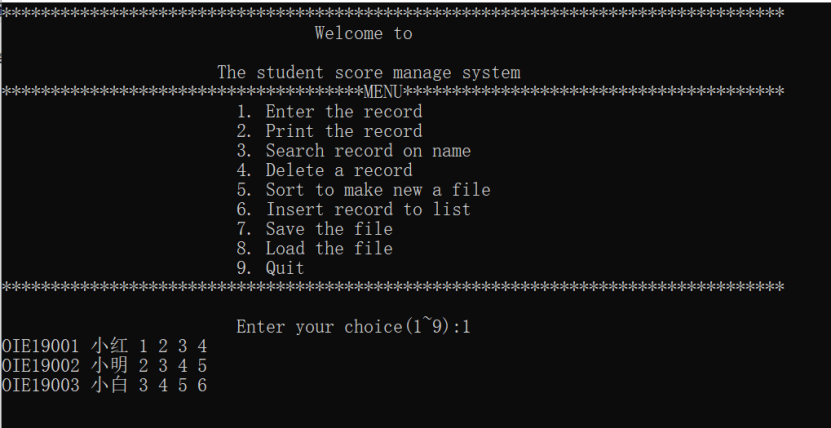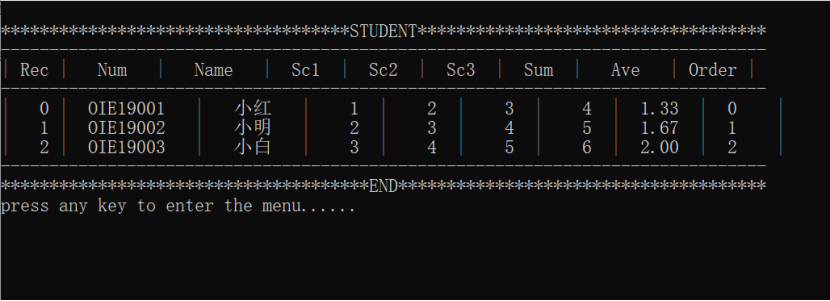查找信息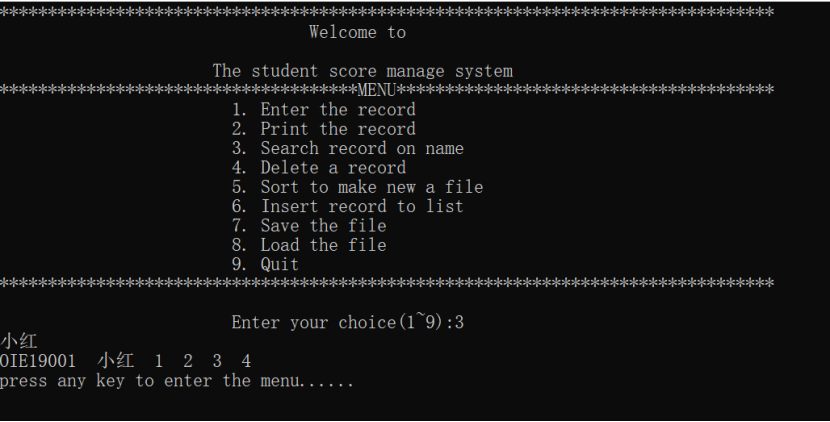插入信息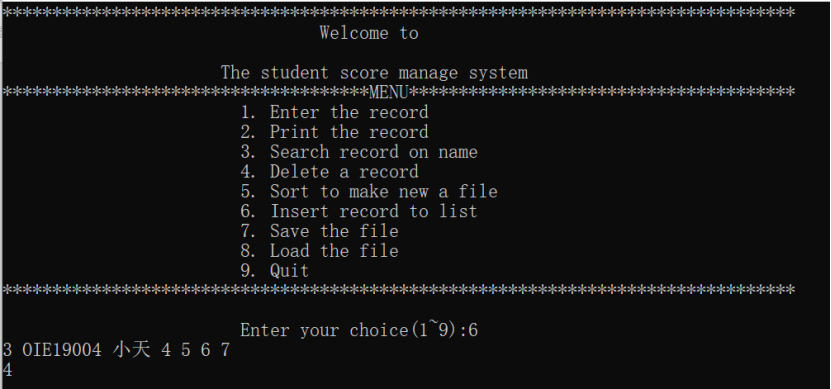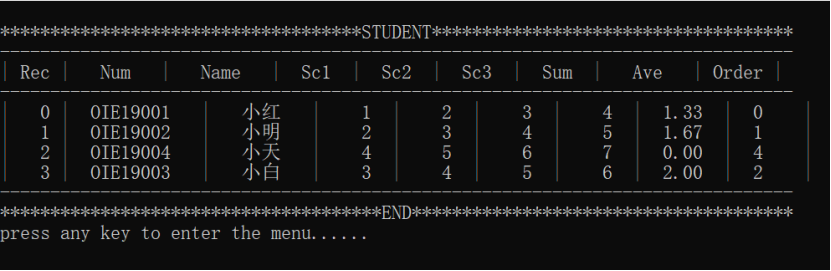删除信息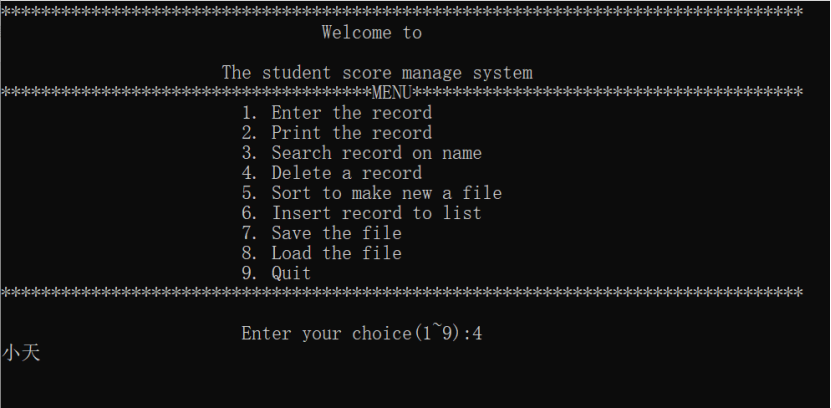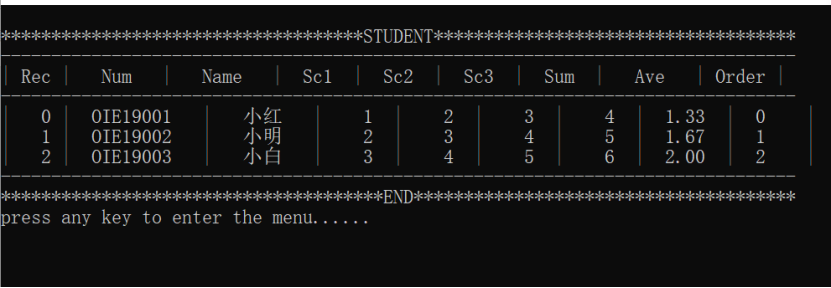排序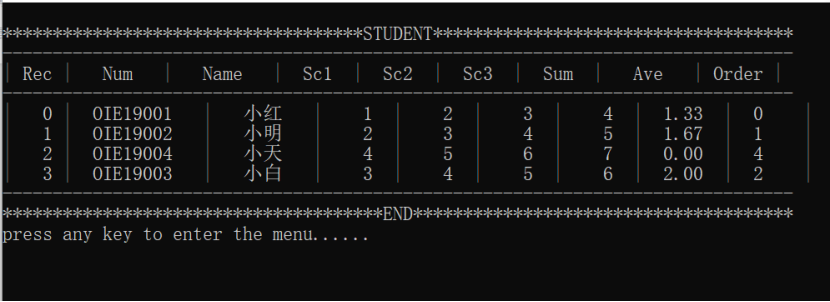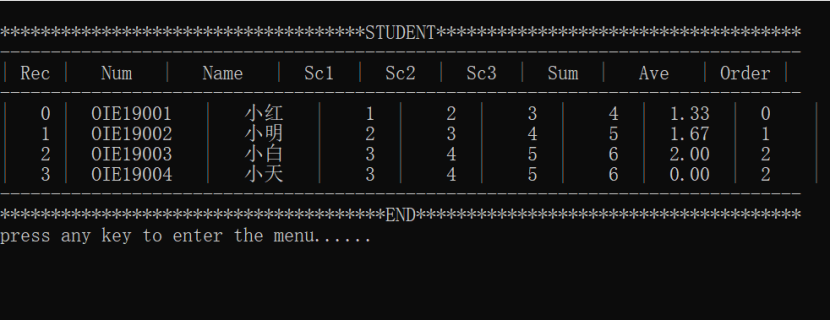//另外这是某学校实践周作业，清楚的同学记得改改代码

展开全文• 主要为大家详细介绍了C语言利用结构体数组实现学生成绩管理系统，具有一定的参考价值，感兴趣的小伙伴们可以参考一下
• 结构体数组 结构体数组格式：声明变量类型 数组名称[数组长度] //定义一个结构体数组名为a,它里面包含有5个元素，每一个元素都是一个结构体变量 Wor a={}; ...

# 结构体数组

#### 结构体数组的定义：

结构体数组格式：声明变量类型 数组名称[数组长度]

//定义一个结构体数组名为a,它里面包含有5个元素，每一个元素都是一个结构体变量
Wor a={};

#include<stdio.h>
typedef struct worker{
char id;
char name;
char sex;
int pay;
}WOR;
void main(){
WOR a=
{
{"0001","陈文杰","男",1200},
{"0001","张三丰","女",500},
{"0001","张四丰","女",1200}
}
}


对比记忆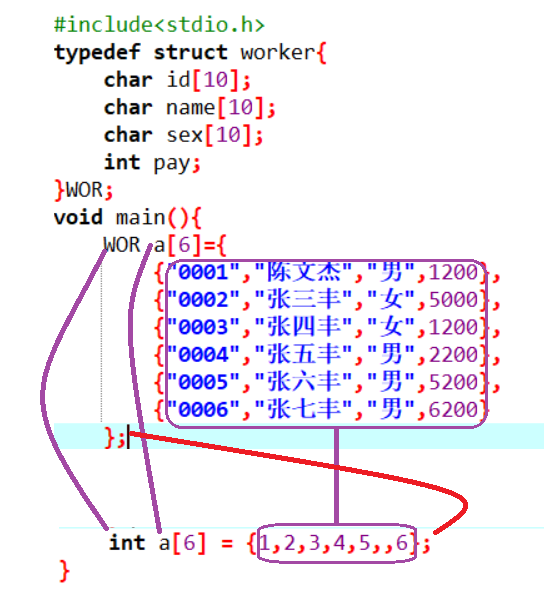#### 结构体数组的输出

#include<stdio.h>
typedef struct worker{
char id;
char name;
char sex;
int pay;
}WOR;
void main(){
WOR a={
{"0001","陈文杰","男",1200},
{"0002","张三丰","女",5000},
{"0003","张四丰","女",1200},
{"0004","张五丰","男",2200},
{"0005","张六丰","男",5200},
{"0006","张七丰","男",6200}
};
int i;
for(i=0;i<6;i++){
printf("%s,%s,%s,%d\n",a[i].id,a[i].name,a[i].sex,a[i].pay);
}
}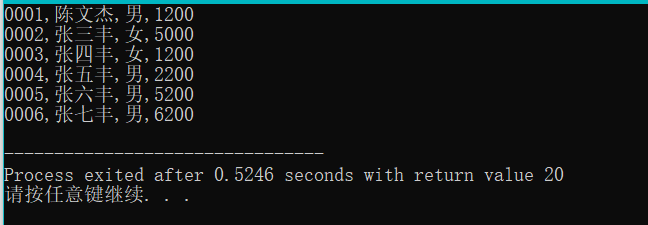结构体对象中的a[i]就代表一个对象，它已经不再是一个值了
所以我们应该用a[ii].成员名

改进以上程序

#include<stdio.h>
typedef struct worker{
char id;
char name;
char sex;
int pay;
}WOR;
void output(WOR a[],int n){//次函数是用来输出长度为n的工人数组的信息
int i;
printf("工号\t姓名\t性别\t年龄\n");
for(i=0;i<6;i++){
printf("%s\t%s\t%s\t%d\n",a[i].id,a[i].name,a[i].sex,a[i].pay);
}
}
void main(){
WOR a={
{"0001","陈文杰","男",1200},
{"0002","张三丰","女",5000},
{"0003","张四丰","女",1200},
{"0004","张五丰","男",2200},
{"0005","张六丰","男",5200},
{"0006","张七丰","男",6200}
};
output(a,6);
}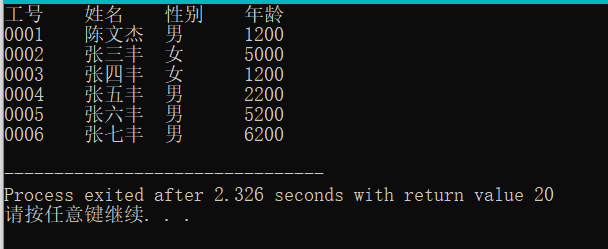#### 结构体的应用

##### 案例一、

1、编写一个函数，它的目的是为了输出所有性别为男的的职工信息
算法分析：
1)这个函数它不用返回值因为该函数是为了完成输出
2）该函数要处理很多职工，就需要一个数组，数组的类型为职工类型
注意：在C语言当中字符串不能直接比较是否相同，要通过strcmp这个函数来比较其两个字符串是否相同

#include<stdio.h>
#include<string.h>
typedef struct worker{
char id;
char name;
char sex;
int pay;
}WOR;
void QueryWorker(WOR a[],int n){//次函数是用来输出长度为n的工人数组的信息
int i;
printf("全部员工：\n");
printf("工号\t姓名\t性别\t年龄\n");
for(i=0;i<6;i++){
printf("%s\t%s\t%s\t%d\n",a[i].id,a[i].name,a[i].sex,a[i].pay);
}
printf("所有男员工：\n");
printf("工号\t姓名\t性别\t年龄\n");
for(i=0;i<6;i++){
if(strcmp(a[i].sex,"男")==0){
printf("%s\t%s\t%s\t%d\n",a[i].id,a[i].name,a[i].sex,a[i].pay);
}
}
printf("所有女员工：\n");
printf("工号\t姓名\t性别\t年龄\n");
for(i=0;i<6;i++){
if(strcmp(a[i].sex,"女")==0){
printf("%s\t%s\t%s\t%d\n",a[i].id,a[i].name,a[i].sex,a[i].pay);
}
}
}
void main(){
WOR a={
{"0001","陈文杰","男",1200},
{"0002","张三丰","女",5000},
{"0003","张四丰","女",1200},
{"0004","张五丰","男",2200},
{"0005","张六丰","男",5200},
{"0006","张七丰","男",6200}
};
QueryWorker(a,6);
}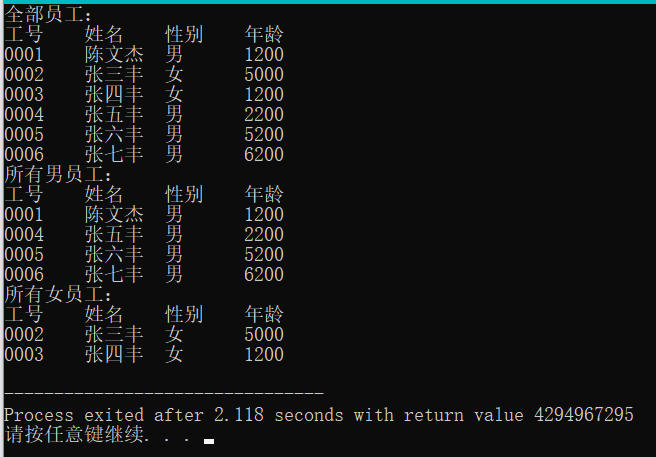2、能够输出指定性别的职工

#include<stdio.h>
typedef struct worker{
char id;
char name;
char sex;
int pay;
}WOR;
void QueryBySex(WOR a[],int n,char b[]){//次函数是用来输出长度为n的工人数组的信息
int i;

printf("工号\t姓名\t性别\t年龄\n");
for(i=0;i<6;i++){
if(strcmp(a[i].sex,b)==0){
printf("%s\t%s\t%s\t%d\n",a[i].id,a[i].name,a[i].sex,a[i].pay);
}
}

}
void main(){
WOR a={
{"0001","陈文杰","男",1200},
{"0002","张三丰","女",5000},
{"0003","张四丰","女",1200},
{"0004","张五丰","男",2200},
{"0005","张六丰","男",5200},
{"0006","张七丰","男",6200}
};
char b;
printf("请输入您要查询的性别 :\n");
scanf("%s",b);
QueryBySex(a,6,b);
}


#### 案例二、

让用户输入一个工号，然后在一个结构体数组当中去查找有没有这个工人，如果有则输出这个工人的信息，如果没有则找不到此人

#include<stdio.h>
typedef struct worker{
char id;
char name;
char sex;
int pay;
}WOR;

void FindById(WOR a[],int n,char id[]){
//我们要访问数组里面每一个工人，如果发现了一个工人，就退出循环。如果找不到就输出找不到此人
int i;
for(i=0;i<n;i++){
if(strcmp(a[i].id,id)==0){//说明第i个工人就是我们要找的职工
break;//强制终止循环
}
}

//在退出循环之后，再来检查i与n的关系
if(i<n){//说明循环在中途就break,发现了职工
printf("%s\t%s\t%s\t%d\n",a[i].id,a[i].name,a[i].sex,a[i].pay);
}
else//找不到职工
{
printf("找不到此人");
}
}

void main(){
WOR a={
{"0001","陈文杰","男",1200},
{"0002","张三丰","女",5000},
{"0003","张四丰","女",1200},
{"0004","张五丰","男",2200},
{"0005","张六丰","男",5200},
{"0006","张七丰","男",6200}
};
char b;
printf("请输入您要查询的id :\n");
gets(b);
FindById(a,6,b);
}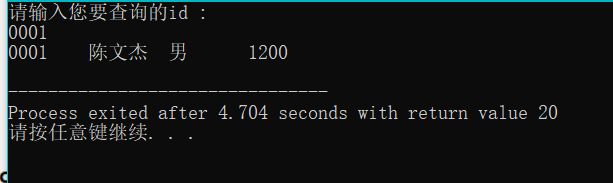#### 案例三、

求出所有职工中最高工资那个职工的信息
注意：这里有一个知识点：成员比较整体赋值

#include<stdio.h>
#include<string.h>
typedef struct worker{
char id;
char name;
char sex;
int pay;
}WOR;
void max(WOR a[],int n){
WOR m=a;
int i;
for(i=0;i<n;i++){
if(m.pay<a[i].pay){//如果m的工资小于a[i]的工资，就把m赋值给a[i]
m=a[i];
}
}
printf("%s\t%s\t%s\t%d\n",m.id,m.name,m.sex,m.pay);
//假设第一个工人是最高工资的那个工人
}
void main(){
WOR a={
{"0001","陈文杰","男",1200},
{"0002","张三丰","女",7000},
{"0003","张四丰","女",1200},
{"0004","张五丰","男",2200},
{"0005","张六丰","男",5200},
{"0006","张七丰","男",6200}
};
max(a,6);
}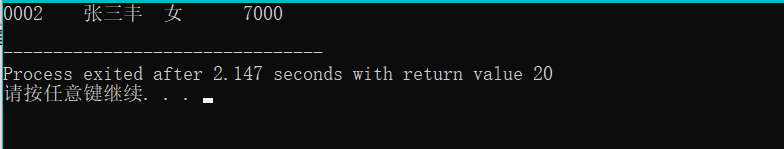上方程序改进
输出所有工资大于平均工资的职工
1、必须要先求出平均
2、在访问每一个职工，如果发现这个职工的工资要小于平均工资的话，就输出这个职工的信息。

#include<stdio.h>
#include<string.h>
typedef struct worker{
char id;
char name;
char sex;
int pay;
}WOR;
void QueryByAver(WOR a[],int n){
int i;
float aver;
int sum = 0;
//求出总工资
for(i=0;i<n;i++){
sum+=a[i].pay;
}
//求出平均工资aver
aver =(float)sum/n;
printf("平均工资为%f\n",aver);
printf("大于平均工资为：\n");
//访问每一个职工
for(i=0;i<n;i++){
if(a[i].pay>=aver){//如果发现职工的工资大于等于平局工资，就输出对应职工的信息
printf("%s\t%s\t%s\t%d\n",a[i].id,a[i].name,a[i].sex,a[i].pay);
}
}
}
void main(){
WOR a={
{"0001","陈文杰","男",3200},
{"0002","张三丰","女",7000},
{"0003","张四丰","女",1200},
{"0004","张五丰","男",2200},
{"0005","张六丰","男",5200},
{"0006","张七丰","男",6200}
};
QueryByAver(a,6);
}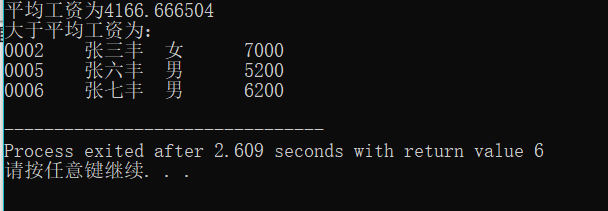算法变换

#include<stdio.h>
#include<string.h>
typedef struct stud{//定义了一个结构体
char Id;//学号
char Name;//姓名
int China;//语文成绩
int Math;//数学成绩
int English;//英语成绩
}STU;
//编写一个函数，它的目的是为了输出所有学生的总分与平均分
void fun(STU a[],int n){
int sum=0,i;
printf("学号\t姓名\t语文\t数学\t英语\t总分\t平局分\n");
//要访问里面的每一个学生
for(i=0;i<n;i++){
//对于一个学生a[i]而言我们要来输出它的信息
printf("%s\t%s\t%d\t%d\t%d\t%d\t%.1f\n",
a[i].Id,a[i].Name,a[i].China,a[i].Math,a[i].English,
a[i].China+a[i].Math+a[i].English,
(a[i].China+a[i].Math+a[i].English)/3.0);
sum = a[i].China+a[i].Math+a[i].English;
}
}
void main(){
STU a={
{"S1","张一军",127,150,100},
{"S2","张二军",75,85,89},
{"S3","张三军",67,58,86},
{"S4","张四军",120,101,87},
{"S5","张五军",136,100,82},
{"S6","张六军",141,52,83},
{"S7","张七军",125,85,81},
{"S8","张八军",136,65,79}
};
fun(a,8);
}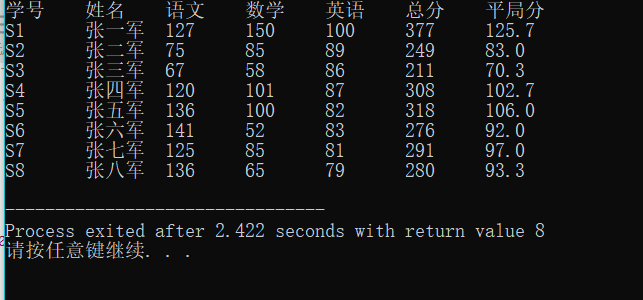案例变换：
注意字符串也不能够直接赋值，要通过一个函数来对字符串进行赋值
strcpy:通过这个函数才可以给字符串进行赋值

#include<stdio.h>
#include<string.h>
typedef struct stud{//定义了一个结构体
char Id;//学号
char Name;//姓名
int China;//语文成绩
int Math;//数学成绩
int English;//英语成绩
}STU;
//编写一个函数，求出每一个学生的优秀科目，目的不是输出分数，而是要输出对应科目的名称
void Perferct(STU a[],int n){
int sum=0,i;
char per;
int maxscore;//用来确保最优科目的分数
//要输出标题
printf("学号\t姓名\t语文\t数学\t英语\t最优秀科目\n");
//要访问里面的每一个学生，对于第i学生要确认它的最优科目名称（语文，数学，英语）
//科目名称是字符串，所以要先定义一个字符串来确保保存最优科目名称
for(i=0;i<n;i++){
maxscore=a[i].China;//认为第i个学生的语文是最优秀的
strcpy(per,"语文");
if(maxscore<a[i].Math){
maxscore = a[i].Math;
strcpy(per,"数学");
}
if(maxscore<a[i].English){
maxscore = a[i].Math;
strcpy(per,"英语");
}
printf("%s\t%s\t%d\t%d\t%d\t%s\n",
a[i].Id,a[i].Name,a[i].China,a[i].Math,a[i].English,per);
}
}

void main(){
STU a={
{"S1","张一军",127,150,100},
{"S2","张二军",75,85,89},
{"S3","张三军",67,58,86},
{"S4","张四军",120,101,87},
{"S5","张五军",136,100,82},
{"S6","张六军",141,52,83},
{"S7","张七军",125,85,81},
{"S8","张八军",136,65,79}
};
Perferct(a,8);
}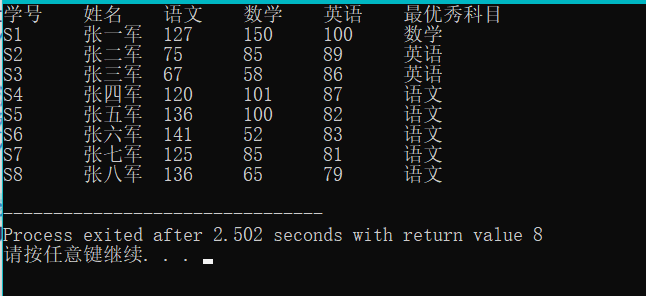展开全文程序设计 指针 编程语言
• 题目描述 定义一个结构体student，存储学生的学号、名字、性别和年龄，读入每个学生的所有信息，保存在结构体中，并输出。...本题要求使用指向结构体数组的指针进行输入输出输入 第一行有一个整数n，表示...

## 题目描述

定义一个结构体student，存储学生的学号、名字、性别和年龄，读入每个学生的所有信息，保存在结构体中，并输出。结构体student的定义如下：
struct student {
int num;
char name;
char sex;
int age;
};
本题要求使用指向结构体数组的指针进行输入和输出。

## 输入

第一行有一个整数n，表示以下有n个学生的信息将会输入。保证n不大于20。
以后的n行中，每一行包含对应学生的学号、名字、性别和年龄，用空格隔开。保证每一个人名都不包含空格且长度不超过15，性别用M和F两个字符来表示。

## 输出

有n行，每行输出一个学生的学号、名字、性别和年龄，用空格隔开。
请注意行尾输出换行。

## 样例输入

3
10101 LiLin M 18
10102 ZhangFun M 19
10104 WangMin F 20


## 样例输出

10101 LiLin M 18
10102 ZhangFun M 19
10104 WangMin F 20

参考代码（有点问题但在oj上可以通过）：

#include<stdio.h>
#include<string.h>

struct student {
int num;
char name;
char sex;
int age;
}stu;

int main()
{
int n;
scanf("%d",&n);
struct student *p;
if(n>=0&&n<=20)
{
for(p=stu; p<stu+n; p++)
{
scanf("%d%s%s%d",&(p->num),&(p->name),&(p->sex),&(p->age));
//ps：scanf里的p->sex必须为%s
}

for(p=stu; p<stu+n; p++)
{
printf("%d %s %c %d\n",p->num,p->name,p->sex,p->age);
//ps：printf里的p->sex必须为%c
}

printf("\n");
}
return 0;
}

ps：输入输出有还有点不太明白，为什么scanf里的p->sex必须为%s，而printf里的p->sex必须为%c才能通过？

若有大佬有幸看到这篇文章，还望指教！！！

## 修改后代码：

#include<stdio.h>
#include<string.h>

struct student {
int num;
char name;
char sex;
int age;
}stu;

int main()
{
int n;
scanf("%d",&n);
struct student *p;
if(n>=0&&n<=20)
{
for(p=stu; p<stu+n; p++)
{
scanf("%d %s %c %d",&(p->num),p->name,&(p->sex),&(p->age));
}
for(p=stu; p<stu+n; p++)
{
printf("%d %s %c %d\n",p->num,p->name,p->sex,p->age);
}
printf("\n");
}
return 0;
}

### ps：当使用scanf进行结构体数组输入时，应当注意字符串类型不需要加取符&。

展开全文• 动态存放学生信息结构体数组，并按成绩排序输出 */ #include <stdio.h> #include <malloc.h> //定义结构体：复杂数据结构 struct Student{ int age; float score; char name; }; //定义...排序算法
• 设计一种结构体，用来保存学生信息。假定学生的总人数不超过100人。 学生信息包括学号(的整数）、姓名（长度不超过19的字符串）、三科成绩（整数）以及综合成绩（实数）除综合成绩外，各项数据均由键盘输入。综合...
• C语言学生高考信息管理。输入输出、排序、查询、插入、删除、修改。
• =40）各学生信息，按平均成绩降序后输出所有女生信息。 1.定义一个学生结构体信息包括学号（字符串，长度小于20）、姓名（字符串，长度小于20）、性别（整数，0表示女生，1表示男生）、年龄（整数）、五门课成绩...
• include<...1.第一种定义结构体的方法 struct student { char name; char idnumber; int score; }; typedef struct studet; 2.第二种定义结构体的方法 typedef struct student { ch...
• 一个结构体变量可以存放一个学生的一组信息，可是如果有 10 个学生呢？难道要定义 10 个结构体变量吗？难道上面的程序要复制和粘贴 10 次吗？很明显不可能，这时就要使用数组。结构体中也有数组，称为结构体数组。它...
• 有3个学生信息，放在结构体数组中，要求输出全部学生信息。 方法：（用指向结构体数组的指针处理） #include<stdio.h> struct Student { int num; char name; char sex; int age; }; struct ...指针
• #include<stdio.h> #define N 10000 ... //大小为4的数组，分别存储4门课程分数 float total; //总分 }; typedef struct student stuStru; /* @函数名称：input 入口参数 ： stuStru s[]...
• C语言结构体数组+结构体类型指针+指向结构体数组的指针+typedef类型结构体数组两种结构体数组的定义形式用sizeof运算符求结构体数组所占字节数结构体类型指针指向结构体数组的指针typedef类型 结构体数组 如果要处理...
• #include<stdio.h> int main() { struct student{ char name; char sex; int age; int num;... }a={{"zhao","nan",12,01},{"wang","nan",14,03},{"guo","nv",11,02}};...i&.
• <p style="text-align:center"><img alt="" src="https://img-ask.csdnimg.cn/upload/1622821894987.jpg" /></p>  </p>
• 实验:学生成绩管理，C语言实现，采用结构体数组完成。 编写程序完成对学生成绩的管理，要求实现的功能包括(以下功能分函数完成): 1、学生信息录入:从键盘...7、学生成绩统计:按分数段统计学生信息采用结构体数组完成。数据结构
• /* ...*All rights reserved. ...*问题描述:输入结构体数组，并根据结构体中的某一数据项整个结构体数组进行排序 *输入描述：； *程序输出： */ #include<stdio.h> #include<string.h> struc...结构体 数组 排序
• 使用选择法进行排序，代码： #include using namespace std; struct Students { int num; char name; char sex; int score; }; int main() { struct Students su ={ {1001,"xway",'M',93},{...
• 题目描述 定义一个结构体student，存储学生的学号、名字、性别和年龄，读入每个学生的所有...本题要求使用指向结构体数组的指针进行输入输出输入 第一行有一个整数n，表示以下有n个学生信息将会输入。保证n不...
• 结构体数组实现学生管理系统，有添加、输出、查找、排序、删除、修改的功能。 #include<stdio.h> #include<string.h> #include<stdlib.h> int n=0; struct student { int num; char name[20...
• 结构体变量以及结构体数组 1、概述 C 语言允许用户自己指定这样一种数据结构，它由不同类型的数据组合成一个整体，以便引用，这些组合在一个整体中的数据是互相联系的，这样的数据结构称为结构体，它相当于其它高级...
• 1/*2动态构造存放学生信息结构体数组，按分数排序输出3*/4#include<stdio.h>5#include<malloc.h>67structStudent8{9intage;10floatscore;11charname;1213};1415intmain(voi...
• 学生信息管理系统中可以用到结构体数组进行数据的输入输出 typedef struct student//定义结构体 { char stu_number; char stu_name; float usual_mark; float exam_mark; float overall_mark; }Student...
• 需要整理三名学生的三科成绩，从键盘上输入结构体数组各元素的值，将结构体数组的内容写入到一个文件中，并从文件中读出，并输出到屏幕上。
• 成绩排名用结构体数组解决，结果输出第一列是名次，注意并列情况；第二列是学号，第三列是成绩。
• [实验案例2：结构体数组]用结构数组建立一张班级成绩单，包括每个学生的学号、姓名、成绩。用函数编程实现下列功能：1. 输入每个学生的各项信息；2. 输出每个学生的各项信息；3. 按照学生的成绩项进行降序排序。...
• ## 结构体和结构体数组

千次阅读 多人点赞 2020-03-14 11:14:21
什么是结构体： ...学校体育考试，Tom得了一个不错的分数，那么请用一个结构体类型保存并输出到控制台 提示：声明student是一个结构体类型；存储如上图一名学生的num（学号），name（姓名）， ...
• 请设计一个结构体存储学生的姓名、学号、英语成绩(ABCD)和数学成绩(百分制)，请录入5个同学的成绩，原样输出后，再按照平均成绩从小到大进行排序后输出。英语成绩转换方法：A-90,B-80,C-70,D-60。 【要求】采用......